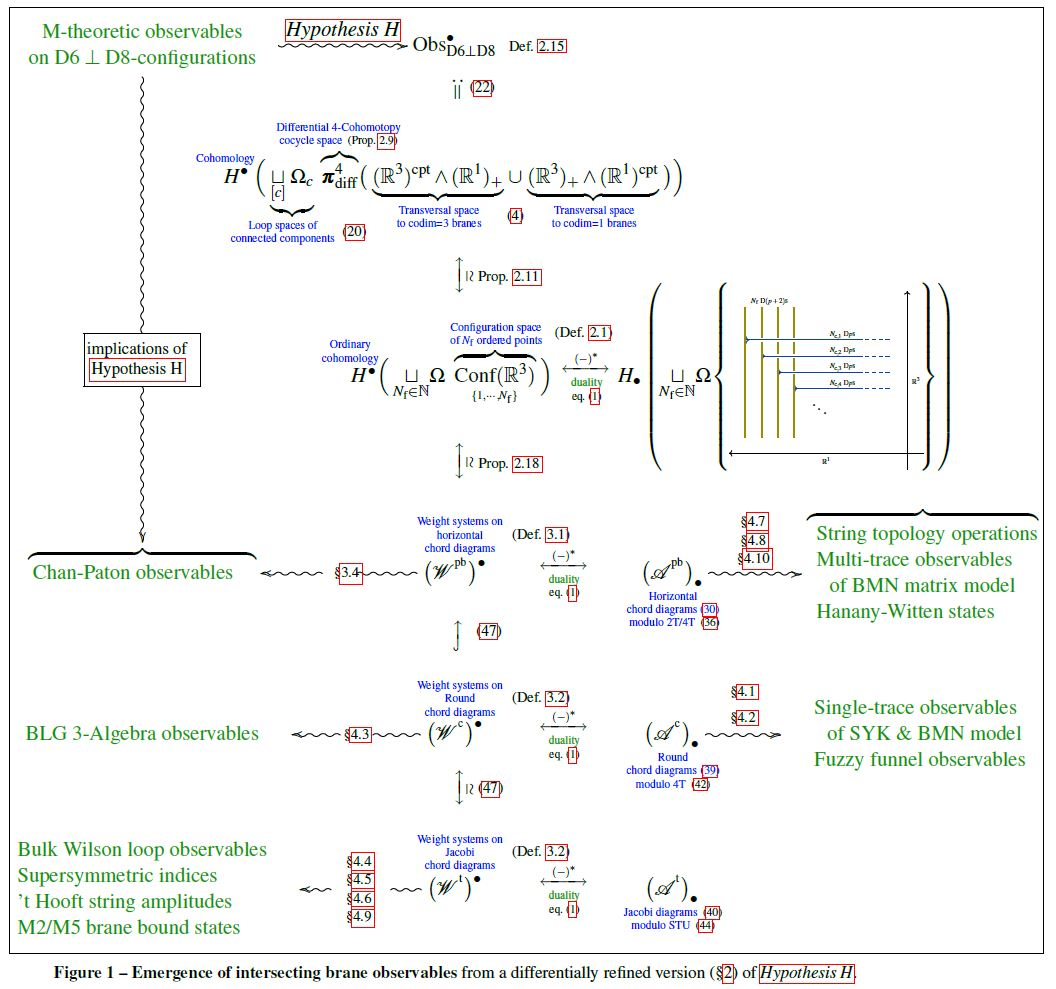# Schreiber Differential Cohomotopy implies intersecting brane observables

an article that we have written:

Hisham Sati, $\;$ Urs Schreiber,

Differential Cohomotopy implies

intersecting brane observables

via configuration spaces and chord diagrams

Advances in Theoretical and Mathematical Physics

26 4 (2022)

ISSN:1095-0753

Abstract: We introduce a differential refinement of Cohomotopy cohomology theory, defined on Penrose diagram spacetimes, whose cocycle spaces are unordered configuration spaces of points. First we prove that brane charge quantization in this differential 4-Cohomotopy theory implies intersecting p/(p+2)-brane moduli given by ordered configurations of points in the transversal 3-space. Then we show that the higher observables on these brane moduli, conceived as the cohomology of the Cohomotopy cocycle space, are given by weight systems on horizontal chord diagrams and reflect a multitude of effects expected in the quantum theory of Dp-D(p+2) brane intersections: condensation to stacks of coincident branes and their Chan-Paton factors, fuzzy funnel states and BLG 3-algebra observables, AdS3-gravity observables supersymmetric indices of Coulomb branches, M2/M5-brane bound states in the BMN matrix model and the Hanany-Witten rules, as well as gauge/gravity duality between all these. We discuss this in the context of the Hypothesis H that the M-theory C-field is charge-quantized in Cohomotopy cohomology theory.Talk notes:

Followup:

Related articles (Hypothesis H)

Last revised on May 24, 2022 at 10:57:48. See the history of this page for a list of all contributions to it.